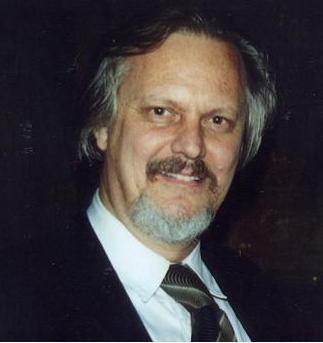Enter the content which will be displayed in sticky bar

# MemberProf. Richard L. Amaroso
local time: 2023-06-10 20:07 (+01:00 )
Prof. Richard L. Amaroso (Abstracts)
Titles
• [Updated 6 years ago]
Abstracts Details
• [Updated 6 years ago]

Many naturally occurring phenomena require theoretical treatment utilizing complex analysis by methods such as the Cauchy-Riemann relations using hyper-geometrical spaces which treat inherently nonlinear, non-dispersive, collective nonlocal resonant states of a quantum system, so as to be consistent with the nonlinearity inherent in General Relativity. Typical quantum approaches form linear approximations limiting the ability to formulate a quantum consistent Relativity Theory. The fundamental nature of remote connectedness is exemplified by Young's double slit experiment, Bell's Theorem, nonlocality, Mach's Principle  and operation of a Foucault pendulum, which may imply the existence of an aether. We demonstrate that a geometric aether is not precluded by the structure of Relativity, although Einstein excluded a fixed reference aether frame. In fact, certain observable phenomena, such as Mach's Principle, Bell's Theorem and Young's double-slit experiment imply the existence of a fixed geometric spacetime aether. A basic tenet of this aether is the quantum principle of nonlocality understood in terms of the soliton-solitary wave solutions of the Schr?dinger equation solved in complex relativistic Minkowski space. Formulation of the complex modified relativistic multidimensional aether allows us to understand the fundamental nature and mechanism of nonlocality allowing experimental designs to further evaluate the properties of nonlocal coherent collective phenomena. The structure of quantum theory using the Schr?dinger equation, covariant Dirac equation and sine-Gordon equation are solved in a complex hyper-eight dimensional relativistic geometric space. The symmetry of this space possesses relativistic Lorentz invariance for nonlinear hyper-dimensional geometry, nonlocality, and nonlinear coherent states which are expanded in terms of quantum soliton solutions.